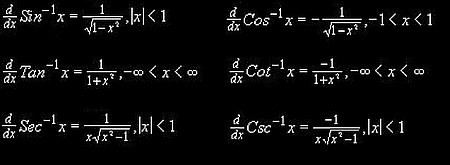# Stewart – Calculus – 3.5 – Implicit Differentiation and Derivatives of Inverse Trigonometric Functions

Find$y''$ by implicit differentiation:$9x^{2}+y^{2}=9$.$18x+2yy'=0$$2yy'=-18x$$y'=-9x/y$$y''=-9(\frac{y\cdot 1\text{--}x\cdot y'}{y^{2}})$$y''=-9(\frac{y\cdot 1\text{--}x(-9x/y)}{y^{2}})$ ($y'$ is replaced with$-9x/y$)$y''=-9(\frac{y^{2}+9x^{2}}{y^{3}})$ (after multiplying by$y$ to eliminate denominator from$-9x$)$y''=-9(\frac{9}{y^{3}})$ (notice that$y^{2}+9x^{2}$ equals the original equation)

So,$y''=\frac{-81}{y^{3}}$.

Derivatives of Inverse Trigonometric Functions